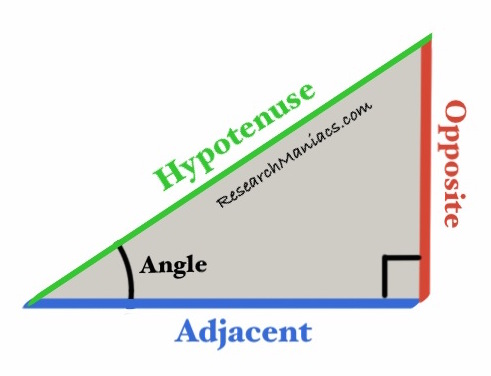What is Tan 11 degrees?Tan is short for tangent. Here we tell you what tan 11 degrees is, how we came up with the answer, and how knowing tan of 11 degrees can be useful.

To calculate tan (11) degrees of a right angled triangle, we use the following equation where angle is 11:

Tan 11 degrees is simply the ratio of the side opposite of the angle to the side adjacent to the angle. As long as the angle stays at 11 degrees, the ratio does not change and tan 11 degrees is a fixed number. See figure 1 for a better illustration. The answer to "What is tan 11 degrees?" is as follows:

Tan(11 deg) ≈ 0.194380309138

Now you also know that the following is true:

This is useful because if you know either Opposite or Adjacent length, you can enter it in the equation above and solve for the missing length.

You can also re-write the equation above to solve for Opposite and Adjacent to get the following new equations:

Figure 1Tan of the angle in the illustration above is calculated by dividing the length of the Opposite by the length of the Adjacent.

If the angle is 11, then the tan of 11 degrees will be 0.194380309138.

Tan Calculator
To find tan of another number, please enter the number below and press "Calculate Tan".

What is Tan 12 degrees?
Go here for the next angle we converted.